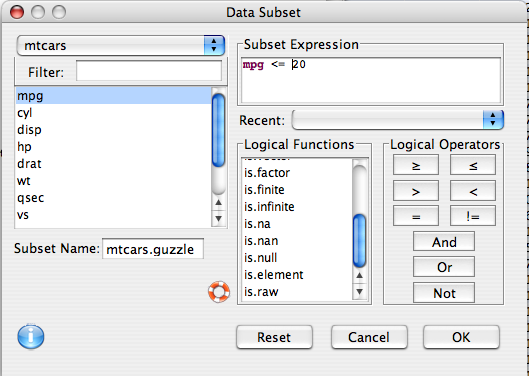## Description

Creates a new data frame that is a subset.

## Dialog

To subset data, choose Subset from the Data menu on the menu bar on the Console window. The following window will appear.Choose the data set from which to create a subset. In this example, the data frame is `mtcars`. Other open files can be chosen from the pull down menu.

Enter the expression for the desired subset in the Subset Expression space. Subset expressions may be formed with any combination of logical comparison operators and functions. Some common ones are listed below. In the example above, the subset was those cars whose miles per gallon were less than or equal to 20 mpg.

After you have entered your subset expression, include a name for the subset in the Subset Name: space. Deducer will automatically provide a name if one is not supplied. Click OK. The subset will appear as a new entry in the pull down menu of the Data Viewer. (The original data remains unchanged.)

Note that to create a subset on non-numeric variables (e.g., type) the values of the variable must be enclosed with quotation marks (e.g., `type == “none”`).

## Common logical operations

 Operator Example Meaning <= mpg<=20 Select observations with mpg less than or equal to 20 >= mpg>=20 Select observations with mpg greater than or equal to 20 < mpg<20 Select observations with mpg less than 20 > mpg>20 Select observations with mpg greater than 20 == cyl==6 Select observations with cyl equal to 6 `!=` cyl!=6 Select observations with cyl not equal to 6 & mpg<=20 & cyl==6 Select observations with mpg less than or equal to 20 and cyl equal to 6 `|` cyl==4 | cyl==6 Select observations with cyl equal to 4 or 6 is.na is.na(cyl) Select observations with missing cyl values `!` `!is.na(cyl)` Select observations with non-missing cyl values

## R Code

`mtcars.guzzle<-subset(mtcars,mpg <= 20)`